# Rhombus angles

If one angle in the rhombus is 159°, what is it neighboring angle in rhombus?

Correct result:

neighboring angle =  21 °

#### Solution:

$\psi =180-159=2{1}^{\circ }$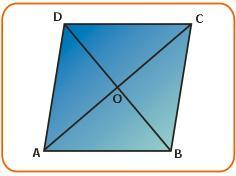We would be pleased if you find an error in the word problem, spelling mistakes, or inaccuracies and send it to us. Thank you!#### You need to know the following knowledge to solve this word math problem:

We encourage you to watch this tutorial video on this math problem:

## Next similar math problems:

• AnglesThe triangle is one outer angle 158°54' and one internal angle 148°. Calculate the other internal angles of a triangle.
• Bicycle wheelAfter driving 157 m bicycle wheel rotates 100 times. What is the radius of the wheel in cm?
• Angles 1It is true neighboring angles have not common arm?
• Complementary angles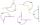Two angles are complementary. If one angle measures 10°, what is the measure of the second angle?
• TrianglesFind out whether given sizes of the angles can be interior angles of a triangle: a) 23°10',84°30',72°20' b) 90°,41°33',48°37' c) 14°51',90°,75°49' d) 58°58',59°59',60°3'
• The angles ratioThe angles in the ABC triangle are in the ratio 1: 2: 3. find the sizes of the angles and determine what kind of a triangle it is.
• Angles in triangle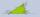Calculate the alpha angle in the triangle if beta is 61 degrees and 98 gamma degrees.
• Ounce749 calories in a 7 - ounce serving . What is the unit rate?
• Parallelogram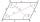In the parallelogram we know one internal angle 67°33`. Calculate the other internal angles.
• I think numberI think number.When I add 841 to it and subtract 157, I get a number that is 22 greater than 996. What number I thinking?
• Outer anglesThe outer angle of the triangle ABC at the A vertex is 71°40 ' outer angle at the vertx B is 136°50'. What size has the inner triangle angle at the vertex C?
• Height of the cylinderThe cylinder volume is 150 dm cubic, the base diameter is 100 cm. What is the height of the cylinder?
• The quotientThe quotient of g and 55 is the same as 279. What is g?
• RatiosReduce the numbers: 50 in a 1:2 ratio 111 at a ratio of 2:3 70 at 10:50 560 at a ratio of 3:8
• Pills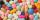If it takes 20 minutes to run a batch of 100 pills how many minutes would it take to run a batch of 50 pills
• Rhombus sidesfind the sides of a rhombus, it area is 550 cm square and altitude is 55cm
• ThalesThales is 1 m from the hole. The eyes are 150 cm above the ground and look into the hole with a diameter of 120 cm as shown. Calculate the depth of the hole.